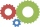# Geometric progression + cube root - math problems

#### Number of problems found: 7

• Annual growthThe population has grown from 25,000 to 33,600 in 10 years. Calculate what was the average annual population growth in%?
• Present valueA bank loans a family \$90,000 at 4.5% annual interest rate to purchase a house. The family agrees to pay the loan off by making monthly payments over a 15 year period. How much should the monthly payment be in order to pay off the debt in 15 years?
• If the 3If the 6th term of a GP is 4 and the 10th is 4/81, find common ratio r.
• Six speedsA drilling machine is to have 6 speeds ranging from 50 to 750 revolutions per minute. If the speed forms a geometric progression, determine their values.
• Three members GPThe sum of three numbers in GP (geometric progression) is 21 and the sum of their squares is 189. Find the numbers.
• Profit growthThe profit of a company increased by 25% during the year 1992, increased by 40% during the year 1993, decreased by 20% in 1994 and increased by 10% during the year 1995. Find the average growth in the profit level over the four years periods?
• Rate or interestAt what rate percent will Rs.2000 amount to Rs.2315.25 in 3 years at compound interest?

We apologize, but in this category are not a lot of examples.
Do you have an exciting math question or word problem that you can't solve? Ask a question or post a math problem, and we can try to solve it.

We will send a solution to your e-mail address. Solved examples are also published here. Please enter the e-mail correctly and check whether you don't have a full mailbox.

Geometric progression - math problems. Cube root - math problems.